# GSEB Solutions Class 12 Physics Chapter 13 Nuclei

Gujarat Board GSEB Textbook Solutions Class 12 Physics Chapter 13 Nuclei Textbook Questions and Answers, Additional Important Questions, Notes Pdf.

## Gujarat Board Textbook Solutions Class 12 Physics Chapter 13 Nuclei

### GSEB Class 12 Physics Nuclei Text Book Questions and Answers

Question 1.
(a) Two stable isotopes of lithium 63Li and 73Li have respective abundances of 7.5% and 92.5%. These isotopes have masses 6.01512 u and 7.01600 u, respectively. Find the atomic mass of lithium.

(b) Boron has two stable isotopes 105B and 115B. Their respective masses are 10.01294 u and 11.00931 u and the atomic mass of boron is 10.811 u. Find the abundances of 105B and 115B.
Solution:
(a) The average atomic mass of lithium(b) Suppose the natural boron contains x% of 105B isotop and (100 – x)% of 115B isotope.
Then atomic mass of natural boron = weighed average of the masses of two isotopes.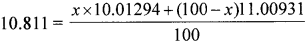On solving, x = 19.9
∴Relative abundance of 105B isotope = 19.9%
∴Relative abundance of 115B isotope = 80.1%

Question 2.
The three stable isotopes of neon: 2010Ne, 2110Ne and 2210Ne have respective abundances of 90.51%, 0.27% and 9.22%. The atomic masses of the three isotopes are 19.99 u, 20.99 u and 21.99 u, respectively. Obtain the average atomic ms of neon.
Solution:
The average atomic mass of neon =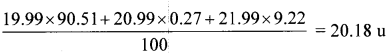Question 3.
Obtain the binding energy (in MeV) of a nitrogen nucleus (147N), given m(147N) =14.00307 u.
Solution:
BE = [{Zmp + (A – Z)mn}-M]c2
Binding energy of 147N = [{7 x 1.007825 + 7 x 1.008665} – 14.00307]931(∴1u = $$\frac{\mathrm{MeV}}{\mathrm{c}^{2}}$$ = 104.61 MeV

Question 4.
Obtain the binding energy of the nuclei Fe and 209131 in units of MeV from the following data.
m(5626Fe) = 55.934939 u, m(20983Bi) 208.980388 u
BE = [(Zm + (A – Z)mn) – M]c2
lu = 931 MeV
∴ BE of 5626Fe = [(26 x 1.007825 + 30 x 1.008665) – 55.934939] 931 MeV = 492 MeV
∴ BE/nucleon =$$\frac {492}{56}$$ = 8.79 MeV
Similarly BE/nucleon of 20983Bi = 7.84 MeVQuestion 5.
A given coin has a mass of 3.0 g. Calculate the nuclear energy that would be required to separate all the neutrons and protons from each other. For simplicity assume that the coin is entirely made of Cu atoms (of mass 62.92960 u).
Solution:
BE = {[Zmp + (A – Z)mn – M} 931 MeV
= [29 x 1.007825 + (63 – 29) x 1 .008665 – 62.92960] 931 = 551.38 MeV
Number of atoms ¡n 63 g of Cu = 6.023 x 1023
∴ Number of atoms in 3 g of Cu = img = 0.2868095 x 1023

∴ The energy required to separate all protons and neutrons,
E = BE x n = 551.38 x 0.2868095 x 1023 = 1.58 x 1025MeV

Question 6.
Write nuclear reaction equations for

1. α – decay of 22688Ra
2. α – decay of 24294pu
3. β – decay of 3215P
4. β – decay of 21083Bi
5. β+ – decay of 116C
6. β+ – decay of 9743Tc
7. Electron capture of 12054Xe.

1. 22688Ra → 22286Rn + 42He
2. 22688Pu → 22286U + 42He
3. 22688P → 22286S + e + $$\overline { \upsilon }$$
4. 22688Bi → 22286Po + e + $$\overline { \upsilon }$$
5. 116C →115B + e+ + υ
6. 116Tc → 116Mo + e+ + υ
7. 12054Xe + e12054I + υ

Question 7.
A radioactive isotope has a half-life of T years. How long will it take the activity to reduce to (a) 3.125%, (b) 1% of its original value?
Solution:
(a) $$\frac{\mathrm{A}}{\mathrm{A}_{0}}$$ = 3 .125% = $$\frac{3.125}{100}$$
$$\frac{\mathrm{A}}{\mathrm{A}_{0}}$$ = $$\frac{100}{3.125}$$ = 32
$$\left(\frac{\mathrm{A}_{0}}{\mathrm{~A}}\right)$$ = 2n i.e., 32 = 2n
n log 2 = 1og 32, n = $$\frac{\log 32}{\log 2}$$ = 5
$$\frac{t}{T}$$ = n = 5, t = 5T

(b) $$\frac{\mathrm{A}}{\mathrm{A}_{0}}$$ = $$\frac{1}{100}$$,
$$\frac{A_{0}}{A}$$ = 100 = 2n
n log 2 = log 100
$$\frac{t}{T}$$ = n = $$\left(\frac{\log 100}{\log 2}\right)$$,
∴ t = 6.65 T

Question 8.
The normal activity of living carbon-containing matter is found to be about 15 decays per minute for every gram of carbon. This activity arises from the small proportion of radioactive 146C present with the stable carbon isotope 126C. When the organism is dead, its interaction with the atmosphere (which maintains the above equilibrium activity) ceases and its activity begins to drop. From the known half-life (5730 years) of 146C, and the measured activity, the age of the specimen can be approximately estimated. This is the principle of 146C dating used in archaeology. Suppose a specimen from Mohen jodaro gives an activity of 9 decays per minute per gram of carbon. Estimate the approximate age of the Indus-Valley civilisation.
Solution:
Normal activity, R0 = 15 decays per min.
Present activity. R = 9 decays per min.
$$\mathrm{T}_{1 / 2}$$ = 5730 days
N = N0e-λt
e-λt = $$\frac{\mathrm{R}}{\mathrm{R}_{0}}$$ = $$\frac{9}{15}$$
λt = loge$$\frac{15}{9}$$ = 2.303 l0g10$$\left(\frac{5}{9}\right)$$ = 0.511
t = $$\frac{0.511}{\lambda}$$ = $$\frac{0.511}{0.693}$$ x $$\mathrm{T}_{1 / 2}$$
= $$\frac{0.511}{0.693}$$ x 5730 = 4225 yearsQuestion 9.
Obtain the amount of 6027Co necessary to provide a radioactive source of 8.0 mCi strength. The half-life of 6027Co is 5.3 years.
Solution:
Activity, A = 8 mCi = 8 x 10-3 Ci = 8 x 10-3 x 3.7 x 1010 = 2.96 x 108s-1
$$\mathrm{T}_{1 / 2}$$ = 5.3 years
5.3 x 365 x 24 x 60 x 60 = 1.67 x 108 s
λ = $$\frac{0.693}{\mathrm{~T}_{1 / 3}}$$ = $$\frac{0.693}{1.67 \times 10^{8}}$$ = 4.15 x 10-9s-1
A = λ N
N = $$\frac{A}{λ}$$ = $$\frac{2.96 \times 10^{8}}{4.15 \times 10^{-9}}$$ = 7.13 x 1016
Mass of 60 g of Co atoms = $$\frac{60 \times 7.13 \times 10^{16}}{6.02 \times 10^{23}}$$ = 7.13 x 1016g

Question 10.
The half-life of 9038Sr is 28 years. What is the disintegration rate of 15 mg of this isotope?
$$\mathrm{T}_{1 / 2}$$ = 28 years = 28 x 365 x 24 x 60 x 60 s = 8.83 x 108 s
Solution:Question 11.
Obtain approximately the ratio of the nuclear radii of the gold isotope 19779Au and the silver isotope 10747Ag.
Solution:
R = R0$$\mathrm{R}_{0} \mathrm{~A}^{1 / 3}$$
For 19779Au, RAu = R0$$(197)^{1 / 3}$$
For 10747Ag, R Ag = R0$$(107)^{1 / 3}$$
$$\frac{\mathrm{R}_{\mathrm{Au}}}{\mathrm{R}_{\mathrm{Ag}}}$$ = $$\left(\frac{197}{107}\right)^{1 / 3}$$ = 1.23

Question 12.
Find the Q – value and the kinetic energy of the emitted α – particle in the α – decay of
(a) 22688Ra (b) 22086Rn
Given m (22688Ra) = 226.02540 u’
m(22286Rn) = 222.01750 u,
m(22086Rn) = 220.01137 u,
m (21684Po) = 216.00189 u.
Solution:
Q = Eα$$\left(\frac{A}{A-4}\right)$$ where Eα is the KE of α particle.
A – mass number of parent
(a) 22688Ra → 22286Ra + α + Q
226.02540 – (222.01750 + 4.002603) = 0.005 5297
∴Q = 0.005297 x 931.5 = 4.934 MeV
∴Eα = 4.934 x $$\frac{A-4}{A}$$ = 4.934 $$\frac{222}{226}$$ = 4.85 MeV

(b) Solve following similar procedure

Question 13.
The radionuclide “C decays according to
116C →115B + e+ + υ, $$\mathrm{T}_{1 / 2}$$ = 20.3 min
The maximum energy of the emitted positron is 0.960 MeV.
Given the mass values: m (116C) = 11.011434 u and m (116B) = 11.009305 u. Calculate Q and compare it with the maximum energy of the positron emitted.
Solution:
The disintegration energy.
Q = (mC – mB – 2mc)c2 = (mC – mB – 2mc) $$\frac{931.5 \mathrm{c}^{2}}{\mathrm{c}^{2}}$$ MeV
2me = 2 x 0.0005486 = 0.0010972Since the maximum energy of positron emitted is 0.960 MeV, the neutrino carries only negligible enegryQuestion 14.
The nucleus 2310Ne decays by β emission. Write down the β-decay equation and determine the maximum kinetic energy of the electrons emitted. Given that:
m (2310Ne) = 22.994466 u
m (2311Na) = 22.089770 u
Solution:
The equation is 2310Ne →2311Na + β + $$\overline { \upsilon }$$ + Q
Q = [mn(2310Ne) + mn2311Na + 10me]c2
(Neglect the rest mass of $$\overline { \upsilon }$$)
= [{mn 2310Ne + 10me} – {mn(2311Na) + 11me}]c2
= [m 2310Ne – m 2311Na]c2
= [22 .994466 – 22.989770]931
= 4.374 MeV

Question 15.
The Q value of nuclear reaction A+ b → C + d is defined by Q = [mA + mb – mC – md]c2 where the masses refer to the respective nuclei. Determine from the given data the Q-value of the following reactions and state whether the reactions are exothermic or endothermic.
I. 11H + 31H→ 21H + 21H
II. 126C +126C – 2010Ne + 42He
Atomic masses are given to be
m (21H) = 2.014102 u
m (31H) = 3.016049 u
m (126C) = 12.000000 u
m (2010Ne) = 19.992439 u
Solution:
I.  11H + 31H→ 21H + 21H
Q = [{m(21H) + m(31H)} – {m(21H) + m( 21H)}]c2
=(4.02387 – 4.028204)931
= – 4.033 MeV (Negative sign shows that the reaction is endothermic)

II. Q [2m(126C) – {m(2010Ne) + m(42He)}]c2
= 2 x 12 – (19.992439 + 4.002603)931
4.618 MeV (exothermic reaction)

Question 16.
Suppose, we think of fission of a 5626Fe nucleus into two equal fragments., 2813Al. is fission energetically possible? Argue by working out Q of the process. Given m ( 5626Fe = 55.93494 u and m (2813Al) = 27.98191 u.
Solution:
Q = [m 5626Fe – 2m(2813Al)]c2
= [55.93494 – 2 x 27.98191]931
= 26.88 MeV (not possible)

Question 17.
The fission properties of 23992Pu are very similar to those of 23992U The average enegry released per fission is 180 MeV. How much energy, in MeV, is released if all atoms in 1 kg of pure 23992Pu undergo fission?
Solution:
Number of atoms in 239 g of 2 23992Pu is Avagadro number.
Number of atoms in 1 g = $$\frac {N}{239}$$ = $$\frac{6.023 \times 10^{23}}{239}$$
Number of atoms in 1 g = $$\frac{6.023 \times 10^{23}}{239}$$ x 103 = 2.52 x 1024 atoms of 23992Pu
Energy released during 1 kg of 23994pu = Energy released by 2.52 x 1024 atoms of 23994Pu
= Energy released per fission x Total number of atoms in 1 kg = 180 x 2.52 x 1024 = 4.536 x 1026 MeV

Question 18.
A 1000 MW fission reactor consumes half of its fuel in 5.00 yrs. how much 23592U did it contain initially? Assume that the reactor operates 80% of the time, that all the energy generated arises from the fission of 23592U and that this nuclei is consumed only by the fission process.
Solution:
The total power generated in 5 yrs. isLet m kg be originally present. Then number of atoms undergone fission is that in $$\frac {m}{2}$$kg.
235 x 10-3 kg contains 6.023 x 1023 atoms.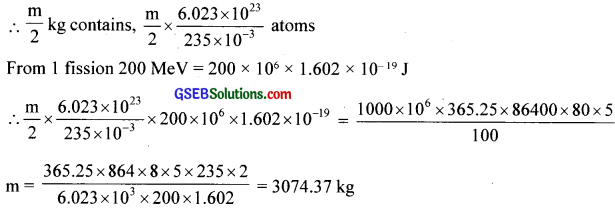Question 19.
How long can an electric lamp of 100 W be kept glowing by fusion of 2.0 kg of deuterium? Take the fusion reaction as
21H + 21H → 32He + n + 3.27 MeV.
Solution:
Mass of deuterium = 2.0141 x 2 = 4.0282 u = 4.0282 x 1.66 x 10-27 kg
No. of fusion in kg of deuterium img = 2.991 x 10-26kg
Energy released per fusion 3.2 MeV = 3.2 x 106 x 1.6 x 10-19 x 2.991 x 1026J
∴ Energy released from 2 kg of 21H = E = 3.2 106 x 1.6 x x 2.991 x j
= 1.5314 x 10-14 J
E = P x tQuestion 20.
For the β+ (positron) emission from a nucleus, there is another competing process known as electron capture (electron from an inner orbit, say, the K-shell, is captured by the nucleus and a neutrino is emitted).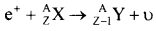Show that if β+ emission is energetically allowed, electron capture is necessarily allowed but not vice – versa.
Solution:
Consider the competing processes:Question 21.
In a periodic table, the average atomic mass of magnesium is given as 24.312 u. The average value is based on their relative natural abundance on earth. The three isotopes and their masses are 2412Mg (23.98504 u), 2512Mg(24.98584 u) and 2612Mg(25.98259 u).
The natural abundance of 2412Mg is 78.99% by mass. Calculate the abundances of other two isotopes.
(2412Mg) = 24.312 u
Mass of 2412Mg = 23.985044
2512Mg = 24.98584 u
2612Mg = 25.98259 u
Let us assume that there are n1 atoms of 125 isotope, n2 atoms of 2nd etc. Total (n1 + n2 + n3) hence (n1 + n2 + n3) 24.312 gives the total mass.
Relative abundance of first = $$\frac{n_{1}}{\left(n_{1}+n_{2}+n_{3}\right)}$$
Relative abundance of 2nd = $$\frac{n_{2}}{\left(n_{1}+n_{2}+n_{3}\right)}$$
Relative abundance of 3rd = $$\frac{n_{3}}{\left(n_{1}+n_{2}+n_{3}\right)}$$
∴ n1 x 23.98504 + n2 x 24.98584 + n3 x 25.98259 =(n1 + n2 + n3) x 24.3124
Sothat $$\frac{n_{1}}{\left(n_{1}+n_{2}+n_{3}\right)}$$ x 23.98504 + $$\frac{n_{2}}{\left(n_{1}+n_{2}+n_{3}\right)}$$ x 24.98584 + $$\frac{n_{3}}{\left(n_{1}+n_{2}+n_{3}\right)}$$ x 25.98259 = 24.3126
$$\frac{n_{1}}{\left(n_{1}+n_{2}+n_{3}\right)}$$= 0.7899.
$$\frac{n_{2}}{\left(n_{1}+n_{2}+n_{3}\right)}$$ = x,
$$\frac{n_{3}}{\left(n_{1}+n_{2}+n_{3}\right)}$$ = 0.2101 – x
∴ 0.7899 x 23.98504 + x x 24.98584 + 0.2101 x 25.98259 – x x 25.98259 = 24.3126
18.9457515 + 5.458942153 – 24.3126 = (25.98259 – 24.98584)x
x = $$\frac{0.092093653}{0.99675}$$ = 0.09239
i.e., 9.24%
Abundance of last isotope = 0.1177
. i.e., 11.77Question 22.
The neutron separation energy is defined as the energy required to remove a neutron from the nucleus. Obtain the neutron separation energies of the nuclei 4120Ca and 2713Al from the following data:
m(4020Ca) = 39.962591 u
m(4120Ca) = 40.962278 u
m(2613Al) = 25.986895 u
m(2713A1) = 26.981541 u
Solution:
Neutron separation energy Sn of a nucleus AzX is given by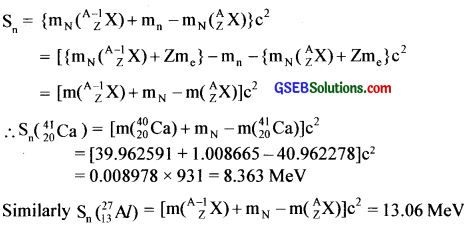Question 23.
A source contains two phosphorus radio nuclides 3215p ($$\mathrm{T}_{1 / 2}$$ = 14.3 d) and 3315p ($$\mathrm{T}_{1 / 2}$$ = 25.3 d). Initially, 10% of the decays come from 3315P . How long one must wait until 90% do so?
Solution: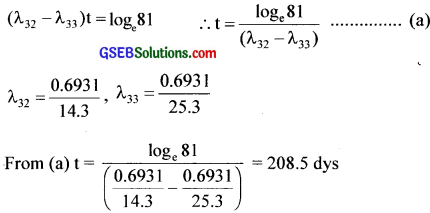Question 24.
Under certain circumstances, a nucleus can decay by emitting a particle more massive than an a -particle. Consider the following decay processes:Calculate the Q-values for these decays and determine that both are energetically allowed.
Solution:Question 25.
Consider the fission of 23892U by fast neutrons. In one fission event, no neutrons are emitted and the final end products, after the beta decay of the primary fragments, are 14058Ce and 9944Ru. Calculate Q for this fission process. The relevant atomic and particle masses are
m(23892U) = 238.05079 u
m(14058Ce) = 139.90543 u
m(9444Ru) = 98.90594 u
Q = [m(23892U) + mn – m(14058Ce) – m(9944Ru)]c2
= [238.05079 + 1.008665 – 139.90543 – 98.90594]931 = 231.1 MeV

Question 26.
Consider the D – T reaction (deuterium – tritium fusion)
21H + 31H → 42He + n

(a) Calculate the energy released in MeV in this reaction from the data:
m(21H) = 2.014102 u
m(31H) = 3.016049 u

(b) Consider the radius of both deuterium and tritium to be approximately 2.0 fm. What is the kinetic energy needed to overcome the coulomb repulsion between the two nuclei? To what temperature must the gas be heated to initiate the
reaction?
(Hint: Kinetic energy required for one fusion event = average thermal kinetic energy available with the interacting particles = 2$$\left(\frac{3 \mathrm{kT}}{2}\right)$$ ; k = Boltzman’s constant, T = absolute temperature.)
Solution:
(a) Q = m(21H) + m(31H) – m(41He) + mn]c2 = (2.014102 + 3.016049 – 4.002603 – 1.008665)931 = 17.59MeV

(b) Repulsive potential energy. ER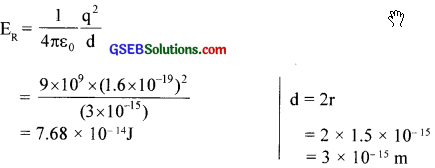Classically, a KE atleast equal to the above amount is required to overcome coulomb repulsion
KE = Ek = 7.68 x 10 -14
KE = $$\frac {3}{2}$$kT = Ek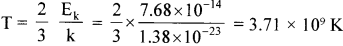Question 27.
Obtain the maximum kinetic energy of f3 particles, and the radiation frequencies of y decays in the decay scheme shown in figure. You are given that
m(198Au) = 197.968233 u
m(198Hg) = 197.966760 u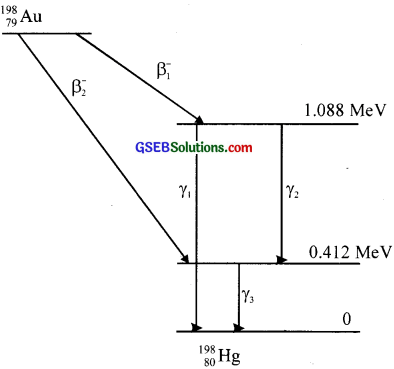Solution: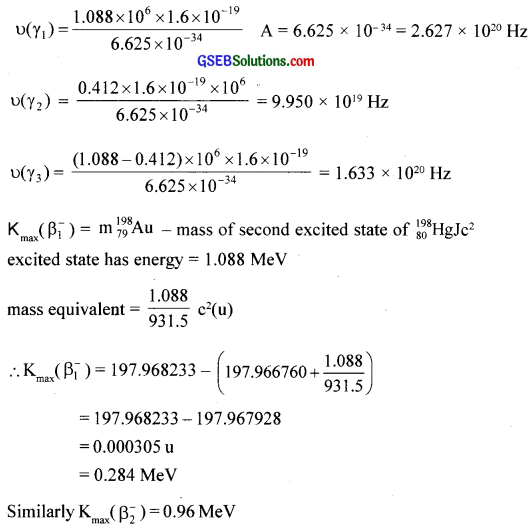Question 28.
Calculate and compare the energy released by (a) fusion of 1.0 kg of hydrogen deep within Sun and (b) the fission of 1.0 kg of 235U in a fission reactor.
Solution:
(a) 1 g of 11H contains Avogadro’s number
∴1kg of 11H contains 6.023 x 1023 x 103
4 11H is required for a reaction, henceQuestion 29.
Suppose india had a target of producing by 2020 AD, 200,000 MW of electric power, ten percent of which was to be obtained from nuclear power plants. Suppose we are given that, on an average, the efficiency of utilization (i.e. conversion to electric energy) of thermal energy produced in a reactor was 25%. How much amount of fissionable uranium would our country need per year by 2020? Take the heat energy per fission of 235U to be about 200MeV.
Solution:
Power required from reactor ~2 x 1010 W
For 1 year it is 2 x 1010) x 365.25 x 86400
Let m kg of uranium is required.
From 1 kg the energy released is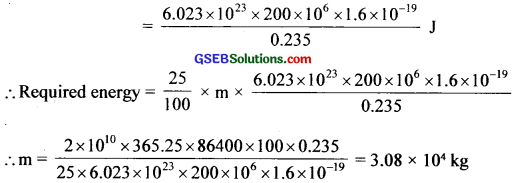Question 1.
How can you find the age of fossils?
Using carbon dating

Question 2.
What is the significance of BE inside the nucleus?
It holds the nucleons together inside the nucleus.

Question 3.
What happens lo the stability of nucleus, when BE increases?
Nucleus becomes more stable.Question 4.
How does nuclear fusion occur. even though coulombic repulsion exists?
Nuclear force is stronger than the Coulombic force.

Question 5.
Nuclear fusion is called thermonuclear reaction. Why?
Since the reaction takes place at a very high temperature of the order of million degree celsius. it is called thermonuclear reaction.

Question 6.
Classify the following statements into true or false.

1. Radioactivity j.c unaffected by pressure and temperature.
2. Radioactivity is affected by large electric and magnetic fields.
3. The rate of disintegration of a radioactive substance at a particular rime is directly proportional to the number of atoms present initially.
4. Nuclear force is charge independent but it depends on the relative orientation of the spins.

1. True
2. False
3. False
4. True

Question 7.
Match the following

 α -Partide Electromagnetic waves Speed is greater than c β – Particle Fast moving neutrons Speed is equal to c γ – rays Nuclei of helium atom Speed is aqual to 0.66c Fast moving electrons Speed is about $$\frac{1}{10}$$ velocity of light

 α -Partide Nuclei of helium atom Speed is about $$\frac{1}{10}$$ velocity of light β – Particle Fast moving electrons Speed is aqualt 0.66c γ – rays Electromagnetic waves Speed is equal to c

Question 8.
(a) Is there any well defined boundary for a nucleus?
(b) What is the order of the size of a nucleus?
(c) How will you conclude that an atom of has a lot of empty space?
(a) No
(b) Fermi
(c) Diameter of atom (including position of electrons) is of the order (≈) of 10-10m and that of
nucleus is 10-15m. The ratio is 105 So much empty space ¡s present.Question 9.(a) What are ‘P ‘ and ‘Q’?
(b) What are the specialties of P?
(a) P-neutron (10n)
Q – energy
(b) i. A free neutron is unstable.
ii. Except hydrogen, it is present in the nucleus of all elements.
iii. Being a changeless particle, it is deflected by electric and magnetic fields.
iv. Energized neutron has high penetrating power.

Question 10.
The radius of a nucleus is R.
(a) How is it related to atomic mass (A)?
(b) Give the relation.
(c) What is the value of the constant of proportionality?
(a) $$\mathrm{R} \propto \mathrm{A}^{\frac{1}{2}}$$
(b) $$\mathrm{R}=\mathrm{R}_{0} \mathrm{~A}^{\frac{1}{3}}$$
(c) R0 = (1.2 ±0.2) x 10-15 m

Question 11.
You have 116C,126C and 136C.
(a) What are these nuclides?
(b) How do they differ?
(a) They are isotopes
(b) In their mass numbers with difference in number of the neutrons.

Question 12.
We have 3919K and 3717Cl.
(a) What are these nuclides?
(b) How do they differ?
(a) They are isotones
(b) May have the same number of neutron but differ in their atomic number and atomic mass.

Question 13.
There are two nuclides 2210Ne and 2211Na.
(a) What are these nuclides?
(b) How do they differ?
(a) They are isobars
(b) Same number of nucleons but different number of protons

Question 14.
What are Isomers? Give examples.
Isomers are nuclides with same number of protons and neutrons, but having different energy states and spins. They have radioactive property.
eg: 99Tc & 99mTc, m – stands for meta stable state & Tc – Technetium

Question 15.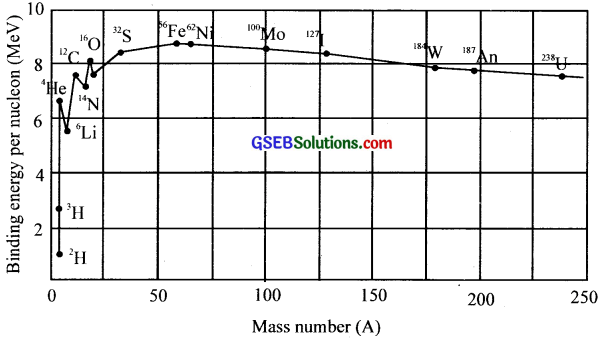(a) What does the graph represent?
(b) What is the importance of this graph?
(c) What does the peak in the graph represent?
(a) Binding energy/nucleon with atomic mass.
(b) It shows the stability of atomic nucleus
(c) The maximum stable condition of an element.Question 16.
In certain isobars the number of protons of one isobar is equal to the number of protons in another.
(a) What is the name given to such isobars?
(b) Give one example.
(a) Isomers
(b) 99Tc & 99mTc

Question 17.
Z. A and M represent the atomic number, mass number and rest mass of a nucleus.
(a) Show that ‘M is always less than the mass of the constituent part ides.
(b) What is this mass difference called?
(c) Give the relation.
(a) Protons and neutrons come together within a very small space of the order of 10-14m to form a nucleus. The energy required to do so is provided by nucleus at the expense of their masses.
Hence the reason.
e.g., For 5B10, the mass, M = 10.012944
5B10 consists of 5 protons and 5 neutrons.
Hence total mass mp + mn
= 5 x Proton mass + 5 x Neutron mass
= 5 x 1.007825 + 5 x 1.008665
= 5.039125 + 5.043325 = 10.08245 u
i.e., M <(mp + mN), hence the reason.

(b) This mass difference is called mass defect (∆m)
(c) ∆m = Zmp + (A – Z)mp – M

Question 18.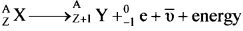(a) What are $${ }_{-1}^{0} \mathrm{e}$$ and $$\bar { \upsilon }$$ ?
(b) What ¡s the name given to this type of emission?
(a) β-particle and anti-neutrino
(b) β-decay

Question 19.
A nuclear even is shown in the diagram.(a) Name the nuclear phenomenon.
(b) What happens to the residual nucleus? Explain.
(c) Compare ¡he binding energy per nucleon of the daughter and the parent.
(d) At what time the hυ is emitted? Explain.
(b) It rebounds (or recoils): due to momentum conservation principle.
(c) The BE per nucleon increases by the activity.
(d) There is no specific time. It is spontaneous. γ -emission can be independent or followed by α – decay

Question 20.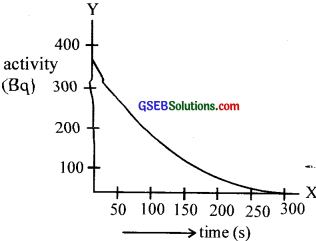The radioactive decay of an element is shown in the graph.
(a) What is the sort of functional decay shown here?
(b) What is the change in activity in equal intervals of time?
(c) Find the half-flfe.
(d) Can you find the decay constant? if so, what is its value?
(a) Hint: Apply λ = $$\frac{0.931}{\mathrm{~T}_{\frac{1}{2}}}$$
(b) Exponential decay
(c) y decreases by the same ratio
(d) Approximately 70 s.Question 21.
Mention the different units of radioactivity
Becquerel (Bq), Curie (Ci), Rutherford (Rf)
1 Bq = 1 disintegration/sec
1 Ci = 3.7 x 1010 disintegration/sec
1 Rf = 106 disintegration/sec

Question 22.
Why are neutrons very effective as bombarding particles?
As neutrons do not have any charge, they are not repelled by nucleus. Hence neutrons are more effective bombardment particles compared to charged particles.

Question 23.(a) What are the different types of emission taking place here?
(b) Name the product after each emission.
(a) α – emission, β – emission and γ – emission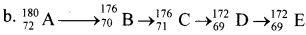(c) When α – emission takes place, atomic number (Z) decreases by 2 and mass number (A) decreases by 4 and we get B. When a 17670B-emission takes place, Z increases by I and A remains the same. Thus we get 17671C. Again an β-emission takes place and we get 17269D.
Lastly γ – emission takes place and there is no change for Z and A. Thus we get 17269E.

Question 24.From the above lubie we can see that the ratio of their atomic masses is 2. But it ¡s not exactly 2, Why is it so? Explain.
Because the mass of a nucleus is slightly less than the mass of the constituent nucleons. (Actual atomic mass is different from mass number)

Question 25.
(a) What is a positron:? b. What is its charge?
(a) A positron is the antiparticle of electron
(b) Same as that of electron but +ve.

Question 26.
What is
i. neutrino
ii. antineutrino?
Explain with examples.
i. -β (i.e.. electron) emission is along with the emission of an antineutrino.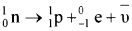ii. +β (positron) emission is along with neutrino.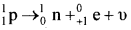Neutrino and antineutrino have no charge and no mass.

Question 27.
α – particles have a high ionizing power. Justify our answer.
α – particles have a charge of + 3.2 x 10-19C and resembles helium nuclues.Question 28.
(a) Are the equations of nuclear reactions such as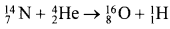‘balanced’ in the sense a chemical equationIf not, in what sense are they balanced on both sides?
(b) If the number of protons and number of neutrons are conserved in a nuclear reaction, in what way the mass’ is converted Into energy (or vice versa) in a nuclear reaction?
(c) A general impress ion exists that mass energy inter-conversion takes place only in nuclear reaction and never in chemical reaction. Strictly speaking, this is incorrect. Explain.
(a) The total number of atoms remains the same in a chemical reaction. The number of atoms of each element is not necessarily conserved in a nuclear reaction.
(b) The binding energy (total) of the elements before the nuclear reaction need not be equal to the total binding energy after the reaction.
(c) There is mass defect in chemical reaction but it is almost a million times smaller compared with nuclear reaction.

Question 29.
Complete the nuclear reaction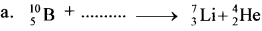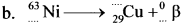Question 30.
(a) Which is more stable, 73Li or 43Li?
(a) 73Li, is-stable
(b) It contains large number of neutrons than the other.

Question 31.
(a) What is the working principle behind
i. Atom bomb
ii. Hydrogen bomb
(b) What is meant by chain reaction?
(c) What happens if it is not controlled?
(d) Is there any device to control it?
(a) i. Atom bomb – Nuclear fission
ii. Hydrogen bomb – Nuclear fusion
(b) Chain reaction is a series of fission process.
(c) A huge amount of energy is released which will lead the world to a disaster.
(d) Yes

Question 32.
(a) Are heavy nuclei stable or unstable?
(b) Give reason.
(a) Unstable
(b) Binding energy per nucleon is small for heavy nuclei.

Question 33.
You are given two nucleoside 73X and 43Y.
(a) Are they the isotopes of the same element?
(b) Which one of the two is likely lo he more stable?
(c) Give reason.
Ans.
(a) Both arc isotopes of the same element since they have the same atomic number.
(b) 73x
(c) Nuclides with greater number of neutrons is stable. So here 43 X is more stable than 73Y.

Question 34.
What is the ratio of nuclear densities of two nucleus having mass numbers in the ratio 1:2?
The nuclear density is independent of mass number. So the ratio is one.Question 35.
(a) Give the expression for the radius of a nucleus.
(b) What is the rutio of the nuclear radii of 19779Au and 10747Ag?
(c) What is the ratio of their nuclear densities?
(a) R = $$\mathrm{R}_{0} \mathrm{~A}^{\frac{1}{3}}$$
(b) $$\mathrm{R}_{0} \mathrm{~A}^{\frac{1}{3}}$$
(c) Registration

Question 36.
(a) is free neutron a stable particle?
(b) Give reason.
(a) No
(b) A free neutron spontaneously decays into a proton, electron and an antineutrino.

Question 37.
(a) Give two radioactive elements which are not formed in observable quantities in nature.
(b) Give reason for this.
(a) Plutonium, Tritium
(b) They have very small half-life period. Hence the reason.

Question 38.
What is positive β – emission?
When a neutron splits into a proton, neutrino and positron, the emission is called positive β emission.

Question 39.
The mechanism of β emission can be stated as a neutron decay. Explain.
A neutron decays into a proton (11H) and an electron (0-1e). β-emission is the emission of electrons from the nucleus.Actually there is an emission of a third particle called anti-neutrino ($$\bar { \upsilon }$$) in the β-emission process, so that both momentum and energy to be conserved.Question 40.
Which raw material is used in a fast breeder reactor?
Thorium

Question 41.
Hermes water is used as a moderator in a nuclear reactor Give reason
Heavy water does not have a tendency to absorb neutrons. It slows down the fast moving neutrons.

Question 42.
What are
(a) slow neutrons?
(b) thermal neutrons?
(c) fast neutrons?
(a) Slow neutrons → energy less than 1 eV
(b) Thermal neutrons → energy range 1 – 1.2 eV
(c) Fast neutrons → energy greater than 1.2 eVQuestion 43.
(a) What factors make a fusion reaction difficult ¡o achieve?
(b) Why does a fusion reactor produce less radioactive waste than a fission reactor?